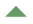```###############################################
# HOW TO USE THIS FILE
# This is an HTML version of the R script file ex4.R
# It is intended to show you the code and to allow links.
# NOT to use interactively to run the code
# to view the ouptut from these commands in html go to R results page```
`red stuff (R commands)`
```#

#
Equal opportunities policies weighted and unweighted - Table 4.3
E O policy by workplace size Table 4.4
Other factors by equal opportunities policy Table 4.5
Logistic regression Table 4.6
Logistic regression Table 4.7
Finite population corrections Table 4.8

# to get names of variables
names(wers)
#
# the ethnic group percentages are in a column labelled ETHNIC
# to get a histogram of this variable do
#
hist(wers\$ETHNIC)
#
#  now it is time to try using the survey package
#  to give R access to the survey functions do - see mini guide if this fails
#
library(survey)

Back to top## ################ table 4.3 ###############################
#
#
# The first step is to create a SURVEY DESIGN OBJECT that
# will contain both the data and the information about the design
#
# This design has WEIGHTS (EST_WT),but to get the correct
# design effects without using the option deff="replace"
# we need to use the weights that add to the population (GROSSWT)
#  and it is stratified  by size of workplace
# and sector. There are 71 strata indexed by STRATA
#  Since it is not clustered we set the sampling unit ids
#  to be the serial number SERNO, different for each workplace
wers.des <- svydesign(id=~SERNO, strata=~STRATA,weights=~GROSSWT,data=wers)
#
#
# now we can look at the survey weighted mean of the proportions
# of workplaces with an EO policy using the svymean command
# giving the design as the second argument to the function
#
svymean(~EO,wers.des)
#
# sadly this just gives a missing value since EO has 7 missing values
# but the answer is to remove the missing data from the survey design
# see the help for is.na and for subset.surveydesign
#
svymean(~EO,subset(wers.des,!is.na(EO)),deff=T)
#
# we can compare this with the unweighted mean had the design been different
#
wers.des <- svydesign(id=~SERNO, strata=~STRATA,data=wers)
svymean(~EO,subset(wers.des,!is.na(EO)),deff=T)
#
Back to top###################Table 4.4##################################
#
# to get the weighted means for subgroups by employment size
# we can use the svyby command to apply svymean to subgroups
# first resetting the design
#
wers.des <- svydesign(id=~SERNO, strata=~STRATA,weight=GROSSWT,data=wers)
svyby(~EO,~NEMPSIZE,subset(wers.des,!is.na(EO)),svymean,keep.var=TRUE)
#
# the warning messages refer to lonely PSUs (see theory section on checking data)
# This means that the standard errors masy be slightly underestimated
#
Back to top#######################Table 4.5########################################
#
# Now we get means of percent female by EO
#
svyby(~FEMALE,~EO,subset(wers.des,!is.na(FEMALE)),svymean)
#
# again the easisest way to get the standard error of
# the difference is with a with svyglm (see help)
#
summary(svyglm(FEMALE~EO,wers.des) )
#
# obviously workplaces with an EO policy have a higher % women
# we can now do similar analyses for % ethnic minority employees
#
svyby(~ETHNIC,~EO,subset(wers.des,!is.na(ETHNIC)),svymean)
summary(svyglm(ETHNIC~EO,wers.des))
#
# like females a bigger effect in the weighted analysis
# but clear evidence of more ethnic minority employees when policy in place
#
# now find weighted percent in each  categories of disabled worker %s
# since DISABGRP is a factor R gives proportions
#
svymean(~DISABGRP,subset(wers.des,!is.na(DISABGRP)))
#
# now means of EO by disabled groups
#
svyby(~EO,~DISABGRP,subset(wers.des,!is.na(DISABGRP) & !is.na(EO)),svymean)
#
# svyglm compares percent in each disabled group category
# between eo and non-eo workplaces
#
summary(svyglm((DISABGRP=='none')~EO,subset(wers.des,!is.na(wers\$DISABGRP) & !is.na(EO))))
summary(svyglm((DISABGRP=='under 3%')~EO,wers.des[!is.na(wers\$DISABGRP),]))
summary(svyglm((DISABGRP=='3%+')~EO,wers.des[!is.na(wers\$DISABGRP),]))
##############################################################
# now the same things for unweighted data
wers.des <- svydesign(id=~SERNO, strata=~STRATA,data=wers)
#
svyby(~FEMALE,~EO,subset(wers.des,!is.na(FEMALE)),svymean)
summary(svyglm(FEMALE~EO,wers.des) )
svyby(~ETHNIC,~EO,subset(wers.des,!is.na(ETHNIC)),svymean)
summary(svyglm(ETHNIC~EO,wers.des))
svymean(~DISABGRP,subset(wers.des,!is.na(DISABGRP)))
svyby(~EO,~DISABGRP,subset(wers.des,!is.na(DISABGRP) & !is.na(EO)),svymean)
summary(svyglm((DISABGRP=='none')~EO,subset(wers.des,!is.na(wers\$DISABGRP) & !is.na(EO))))
summary(svyglm((DISABGRP=='under 3%')~EO,wers.des[!is.na(wers\$DISABGRP),]))
summary(svyglm((DISABGRP=='3%+')~EO,wers.des[!is.na(wers\$DISABGRP),]))
Back to top##########################################################
# Table 4.6
# now the svyglm command and the glm commands to
# fit logistic regressions
#  first restore design
wers.des <- svydesign(id=~SERNO, strata=~STRATA,weights=~GROSSWT,data=wers)
summary(svyglm(EO~ETHNIC+FEMALE+DISABGRP,wers.des,family='binomial'))
#
# and unweighted
#
wers.des <- svydesign(id=~SERNO, strata=~STRATA,data=wers)
summary(svyglm(EO~ETHNIC+FEMALE+DISABGRP,wers.des,family='binomial'))
Back to top##################################################################
# table 4.7
# now explore models that include workplace size
wers.des <- svydesign(id=~SERNO, strata=~STRATA,weights=~GROSSWT,data=wers)
summary(svyglm(EO~ETHNIC+FEMALE+DISABGRP+NEMPSIZE,wers.des,family='binomial'))
#
# and without weighting
#
wers.des <- svydesign(id=~SERNO, strata=~STRATA,data=wers)
summary(svyglm(EO~ETHNIC+FEMALE+DISABGRP+NEMPSIZE,wers.des,family='binomial'))
Back to top###############################################################
#
#  table 4.8 with finite population corrections
#
wers.des <- svydesign(id=~SERNO,fpc=~SAMPFRAC, strata=~STRATA,weight=~GROSSWT,data=wers)
svymean(~EO,subset(wers.des,!is.na(EO)))
svyby(~EO,~NEMPSIZE,subset(wers.des,!is.na(EO)),svymean,keep.var=TRUE)
#
# and without
wers.des <- svydesign(id=~SERNO, strata=~STRATA,weight=~GROSSWT,data=wers)
svymean(~EO,subset(wers.des,!is.na(EO)))
svyby(~EO,~NEMPSIZE,subset(wers.des,!is.na(EO)),svymean,keep.var=TRUE)
#
#############################################################
# now some more modelling stuff to make plots
#
wers.des <- svydesign(id=~SERNO, strata=~STRATA,weights=~GROSSWT,data=wers)
# disabled now dropped out
#
summary(svyglm(EO~ETHNIC+FEMALE+NEMPSIZE+ETHNIC,wers.des,family='binomial'))

# now  make a factor to test linearity of percent female
#
wers.des<-update( wers.des,GPFEMALE=factor(ceiling(FEMALE/20)*(FEMALE>0)+(1*FEMALE==0),
labels=c('0-<20','20-<40','40-<60','60-<80','80-100') ) )
summary(svyglm(EO~ETHNIC+GPFEMALE+NEMPSIZE,wers.des,family='binomial'))
#
# Linearity for grouped size looks not too bad
# But there seems to be steep increase for workplaces with >50%
# female. For robustness, stick with this model where we fit both of these as categories
#
# fit as simple (not survey) glm
#
#
# fit as simple (not survey) glm
#
summary(glm(EO~ETHNIC+GPFEMALE+NEMPSIZE,wers,family='binomial'))
#
# we can see that the effect of % of female employees is less marked in the bigger workplaces
#  note also that the efect of ethnic group still stands out  clearly in these
# analyses with the odds of EO increasing by around 1.8 (exp(0.3*20)) when the
# percentage ethnic goes from 0 to 20%.
#
# This final bit of code makes a plot of %EO by FEMALE and SIZE to understand findings.
#

wers\$GPFEMALE=factor(ceiling(wers\$FEMALE/20)*(wers\$FEMALE>0)+(1*wers\$FEMALE==0),
labels=c('0-<20','20-<40','40-<60','60-<80','80-100') )
forpred<-data.frame(NEMPSIZE=rep(0:5,5),ETHNIC=rep(2,30),
FEMALE=rep(c(10,30,50,70,90),rep(6,5)))
forpred\$GPFEMALE=factor(ceiling(forpred\$FEMALE/20)*(forpred\$FEMALE>0)+(1*forpred\$FEMALE==0),
labels=c('0-<20','20-<40','40-<60','60-<80','80-100') )
forpred\$NEMPSIZE<-factor(forpred\$NEMPSIZE,labels=c('10-24','25-49','50-99','100-199',
'200-499','500+'))

wt.fit<-predict(svyglm(EO~ETHNIC+GPFEMALE+NEMPSIZE,wers.des,family='binomial'),forpred)
unwt.fit<-predict(glm(EO~ETHNIC+GPFEMALE+NEMPSIZE,wers,family='binomial'),forpred)

invlogit<-function(x){1/(1+exp(-x))}
par(mar=c(5,5,1,1),cex.axis=.9,cex.lab=.9,oma=c(0,0,0,0))
plot(c(0,5),c(40,100),type='n',ylab='% with eo policy',xlab='workplace size group')
for (i in 1:5) points(0:5,100*invlogit(wt.fit)[1:6+(i-1)*6],pch=1,lty=1,type='b',col=i,lwd=2)
for (i in 1:5) points(0:5,100*invlogit(unwt.fit)[1:6+(i-1)*6],pch=2,lty=2,type='b',col=i,lwd=2)

legend(3.3,67,c('10% female' ,'30% female',' 50% female', '70% female',' 90% female'),col=1:5,pch=rep(1,5),lty=rep(1,5),bty='n',cex=.8)
legend(4.5,67,c('' ,'',' ', '',''),col=1:5,pch=rep(2,5),lty=rep(2,5),bty='n',cex=.8)
text(3.5,69,'weighted',cex=.8)
text(4.7,69,'unweighted',cex=.8)
#################################################################
```